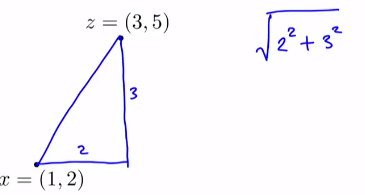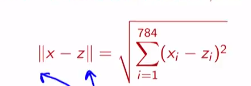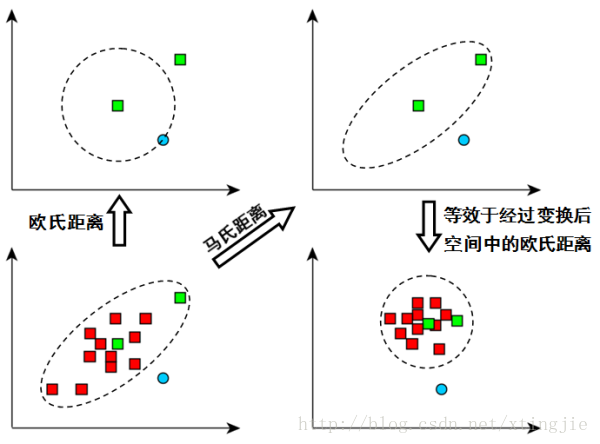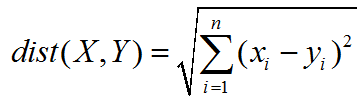|x| = √( x2 + x2 + … + x[n]2 )

m维空间中两个点之间的真实距离

Euclidean Metric，Euclidean Distance

• ## 欧式距离

千次阅读 2019-04-25 20:11:01
二维空间的欧式距离： 高维空间的欧式距离： 这个符号（两个竖线） 在没有特殊说明的情况下表示欧式距离欧式距离衡量了两个向量在每个维度上的差距。计算的方式为： 将每个维度差的平方求和再相加后开方...
二维空间的欧式距离：高维空间的欧式距离：这个符号（两个竖线） 在没有特殊说明的情况下表示欧式距离。欧式距离衡量了两个向量在每个维度上的差距。计算的方式为： 将每个维度差的平方求和再相加后开方。

二维空间的欧式距离：

这个符号（两个竖线） 在没有特殊说明的情况下表示欧式距离。欧式距离衡量了两个向量在每个维度上的差距。计算的方式为： 将每个维度差的平方求和再相加后开方。

展开全文• ## 马氏距离和欧式距离详解

万次阅读 多人点赞 2019-03-28 10:09:02
一般在机器学习模型中会涉及到衡量两个样本间的距离，如聚类、KNN，K-means等，使用的距离为欧式距离。其实，除了欧氏距离之外，还有很多的距离计算标准，本文主要介绍欧氏距离和马氏距离。 欧氏距离 最常见的两点...
一般在机器学习模型中会涉及到衡量两个样本间的距离，如聚类、KNN，K-means等，使用的距离为欧式距离。其实，除了欧氏距离之外，还有很多的距离计算标准，本文主要介绍欧氏距离和马氏距离。

欧氏距离
最常见的两点之间或多点之间的距离表示法，又称之为欧几里得度量，它定义于欧几里得空间中，如点 $x = (x_1,…,x_n)$ 和 $y = (y_1,…,y_n)$ 之间的距离为：
$d(x,y) = \sqrt{(x_1-y_1)^2+(x_2-y_2)^2+...+(x_n-y_n)^2} = \sqrt{\sum_{i=1}^{n}(x_i-y_i)^2}$

二维平面上两点a(x1,y1)与b(x2,y2)间的欧氏距离：
$d_{12} = \sqrt{(x_1-x_2)^2+(y_1-y_2)^2}$

两个n维向量$a(x_{11},x_{12},…,x_{1n})$与 $b(x_{21},x_{22},…,x_{2n})$间的欧氏距离：
$d_{12} = \sqrt{\sum_{k=1}^{n}(x_{1k}-x_{2k})^2}$

马氏距离
在介绍马氏距离之前，我们先来看如下几个概念：

方差：方差是标准差的平方，而标准差的意义是数据集中各个点到均值点距离的平均值。反应的是数据的离散程度。

协方差： 标准差与方差是描述一维数据的，当存在多维数据时，我们通常需要知道每个维数的变量中间是否存在关联。协方差就是衡量多维数据集中，变量之间相关性的统计量。比如说，一个人的身高与他的体重的关系，这就需要用协方差来衡量。如果两个变量之间的协方差为正值，则这两个变量之间存在正相关，若为负值，则为负相关。

协方差矩阵： 当变量多了，超过两个变量了。那么，就用协方差矩阵来衡量这么多变量之间的相关性。假设 $X$ 是以 $n$个随机变数（其中的每个随机变数是也是一个向量，当然是一个行向量）组成的列向量：
$X = \begin{bmatrix} X_1 \\ X_2 \\ \vdots \\ X_n \end{bmatrix}$
其中，$μ_i$是第i个元素的期望值，即$μ_i=E(X_i)$。协方差矩阵的第$i,j$项（第$i,j$项是一个协方差）被定义为如下形式：
$\sum_{ij} = cov(X_i,X_j = E[(X_i-\mu_i)(X_j-\mu_j)])$
即：
$\sum = \begin{bmatrix} E[(X_1-\mu_1)(X_1-\mu_1)]) & E[(X_1-\mu_1)(X_2-\mu_2)]) & \cdots & E[(X_1-\mu_1)(X_n-\mu_n)]) \\ E[(X_2-\mu_2)(X_1-\mu_1)]) & E[(X_2-\mu_2)(X_2-\mu_2)]) & \cdots & E[(X_2-\mu_2)(X_n-\mu_n)]) \\ \vdots & \vdots & \ddots & \vdots \\ E[(X_n-\mu_n)(X_1-\mu_1)]) & E[(X_n-\mu_n)(X_2-\mu_2)]) & \cdots & E[(X_n-\mu_n)(X_n-\mu_n)]) \end{bmatrix}$
矩阵中的第 $(i,j)$ 个元素是 $X_i$ 与 $X_j$ 的协方差。

马氏距离的定义：
马氏距离（Mahalanobis Distance）是由马哈拉诺比斯（P. C. Mahalanobis）提出的，表示数据的协方差距离。它是一种有效的计算两个未知样本集的相似度的方法。与欧氏距离不同的是它考虑到各种特性之间的联系（例如：一条关于身高的信息会带来一条关于体重的信息，因为两者是有关联的）并且是尺度无关的（scale-invariant），即独立于测量尺度。
对于一个均值为$μ=(μ_1,μ_2,μ_3,...,μ_p)^T$，协方差矩阵为$S$的多变量$x=(x_1,x_2,x_3,...,x_p)^T$，其马氏距离为：
$D_M(x) = \sqrt{(x-\mu)^T {S}^{-1}(x-\mu)}$
我们可以发现如果$S^{-1}$是单位阵的时候，马氏距离简化为欧氏距离。
那我们为什么要用马氏距离呢？
马氏距离有很多优点： 马氏距离不受量纲的影响，两点之间的马氏距离与原始数据的测量单位无关；由标准化数据和中心化数据(即原始数据与均值之差）计算出的二点之间的马氏距离相同。马氏距离还可以排除变量之间的相关性的干扰。
下面我们来看一个例子：
如果我们以厘米为单位来测量人的身高，以克（g）为单位测量人的体重。每个人被表示为一个两维向量，如一个人身高173cm，体重50000g，表示为（173,50000），根据身高体重的信息来判断体型的相似程度。
我们已知小明（160,60000）；小王（160,59000）；小李（170，60000）。根据常识可以知道小明和小王体型相似。但是如果根据欧几里得距离来判断，小明和小王的距离要远远大于小明和小李之间的距离，即小明和小李体型相似。这是因为不同特征的度量标准之间存在差异而导致判断出错。
以克（g）为单位测量人的体重，数据分布比较分散，即方差大，而以厘米为单位来测量人的身高，数据分布就相对集中，方差小。马氏距离的目的就是把方差归一化，使得特征之间的关系更加符合实际情况。
下图（a）展示了三个数据集的初始分布，看起来竖直方向上的那两个集合比较接近。在我们根据数据的协方差归一化空间之后，如图（b），实际上水平方向上的两个集合比较接近。深入分析：
当求距离的时候，由于随机向量的每个分量之间量级不一样，比如说x1可能取值范围只有零点几，而x2有可能时而是2000，时而是3000，因此两个变量的离散度具有很大差异
马氏距离除以了一个方差矩阵，这就把各个分量之间的方差都除掉了，消除了量纲性，更加科学合理。如上图，看左下方的图，比较中间那个绿色的和另外一个绿色的距离，以及中间绿色到蓝色的距离
如果不考虑数据的分布，就是直接计算欧式距离，那就是蓝色距离更近
但实际上需要考虑各分量的分布的，呈椭圆形分布
蓝色的在椭圆外，绿色的在椭圆内，因此绿色的实际上更近
马氏距离除以了协方差矩阵，实际上就是把右上角的图变成了右下角
参考资料：
马氏距离通俗理解


展开全文机器学习
• 目录 ...欧式距离源自N维欧氏空间中两点x1,x2x1,x2x_1,x_2间的距离公式： d=∑i=1N(x1i−x2i)2‾‾‾‾‾‾‾‾‾‾√d=∑i=1N(x1i−x2i)2d = \sum_{i=1}^N \sqrt{(x_{1i}-x_{2i})^2} 2.标准化...
目录
欧氏距离
标准化欧氏距离
马氏距离
夹角余弦距离
汉明距离
曼哈顿(Manhattan)距离
1.欧式距离
欧式距离源自N维欧氏空间中两点x1,x2x_1,x_2间的距离公式：d=∑i=1N(x1i−x2i)2d =  \sqrt{\sum_{i=1}^N(x_{1i}-x_{2i})^2}
2.标准化欧式距离（Standardized Euclidean distance）
引入标准化欧式距离的原因是一个数据xix_i的各个维度之间的尺度不一样。
【对于尺度无关的解释】如果向量中第一维元素的数量级是100，第二维的数量级是10，比如v1=(100,10),v2 = (500,40)，则计算欧式距离d=100⋅(5−1)2+10∗(4−1)2d = \sqrt{100\cdot(5-1)^2+10*(4-1)^2}
可见欧式距离会给与第一维度100权重，这会压制第二维度的影响力。对所有维度分别进行处理，使得各个维度的数据分别满足标准正态分布：xi′=xi−uisi x'_i = \frac{x_i-u_i}{s_i}uiu_i是该维度所有数据的均值，sis_i是对应方差。
然后在对x′x'进行欧式距离：d=∑i=1N(xi−yi)2si2d = \sqrt{\sum_{i =1}^N \frac{(x_i-y_i)^2}{s_i^2}}
网络上的Normalized Euclidean distance和Standard Euclidean distance的定义对应这两种处理。
3.马氏距离
马氏距离又称为数据的协方差距离，它是一种有效的计算两个未知样本集的相似度的方法。马氏距离的结果也是将数据投影到N(0,1)区间并求其欧式距离，与标准化欧氏距离不同的是它认为各个维度之间不是独立分布的，所以马氏距离考虑到各种特性之间的联系。
假设uxu_x为向量X={x1,x2,...,xN}X = \{x_1,x_2,...,x_N\}的均值，uyu_y为Y={y1,y2,...yN}Y = \{y_1,y_2,...y_N\}的均值，Σ\Sigma 是X与Y的协方差
点X与Y的马氏距离：(x−ux)TΣ−1(y−uy)\sqrt{(x-u_x)^T\Sigma^{-1}(y-u_y)}
这个式子可以用矩阵的迹来重写：(x−ux)TΣ−1(y−uy)=tr(Σ−1(y−uy)(x−ux)T\sqrt{(x-u_x)^T\Sigma^{-1}(y-u_y)}=\sqrt{tr(\Sigma ^{-1} (y-u_y)(x-u_x)^T}
其中Σ\Sigma是X与Y的协方差矩阵
可见：
如果Σ\Sigma是单位矩阵，则马氏距离退化成欧式距离；
如果Σ\Sigma是对角矩阵，则称为归一化后的欧式距离。
所以马氏距离的特点：
尺度无关
考虑进数据之间的联系
马氏距离可以通过协方差自动生成相应的权重，而使用逆则抵消掉这些权重。
最典型的就是根据距离作判别问题，即假设有n个总体，计算某个样品X归属于哪一类的问题。此时虽然样品X离某个总体的欧氏距离最近，但是未必归属它，比如该总体的方差很小，说明需要非常近才能归为该类。对于这种情况，马氏距离比欧氏距离更适合作判别。
4.余弦距离（余弦相似性）
严格来讲余弦距离不是距离，而只是相似性。其他距离直接测量两个高维空间上的点的距离，如果距离为0则两个点“相同”；余弦的结果为在[0，1][0，1]之中，如果为 1，只能确定两者完全相关、完全相似。
假设两用户同时对两件商品评分，向量分别为(3,3)和(5,5)，这两位用户对两件商品的喜好其实是一样的，余弦距离此时为1，欧式距离给出的解显然没有余弦值直观。
相对于标准化后的欧式距离，余弦距离少了将数据投影到一个均值为0的区间里这一步骤。对于点X和点Y，其余弦距离：d=∑i=1Nxiyi∑iNxi2∑iNyi2d = \frac{\sum_{i=1}^{N}x_iy_i}{\sqrt{\sum_i^N {x_i^2}}\sqrt{\sum_i^N y_i^2}}
余弦距离在给文本分类的词袋模型中使用，例如给一篇文章一共出现过6000个词，则用一个6000维度的向量X表示这篇文章，每个维度代表各个字出现的数目；另外一篇文章也恰好只出现过这6000字，用向量Y表示该文章，则这两篇文章相似度可以用余弦距离来测量。
优点：余弦距离根据向量方向来判断向量相似度，与向量各个维度的相对大小有关，不受各个维度直接数值影响。
某种程度上，归一化后的欧氏距离和余弦相似性表征能力相同。
5.汉明距离
汉明距离是两个等长字符串之间的汉明距离是两个字符串对应位置的不同字符的个数。比如：
1011101 与 1001001 为 2
2143896 与 2233796 是 3
可以把它看做将一个字符串变换成另外一个字符串所需要替换的字符个数。
此外，汉明重量是字符串相对于同样长度的零字符串的汉明距离，如：
11101 的汉明重量是 4。
所以两者间的汉明距离等于它们汉明重量的差a-b
6.曼哈顿距离 (Manhattan  distance）
曼哈顿距离的定义如下：∑p∣∣I1p−I2p∣∣\sum_p||I_1^p-I_2^p||
p是I的维度。当I为图像坐标时，曼哈顿距离即是x,y坐标距离之和。
【补充】协方差矩阵的逆Σ−1\Sigma^{-1}的求法：
先将Σ\Sigma进行SVD分解(由于协方差矩阵是对称矩阵，因此此处严格说来是特征值分解EVD)。
由于协方差矩阵是对称的，UΛVT=Σ=ΣT=(UΛVT）T=VΛUTU\Lambda V^T = \Sigma = \Sigma^T =(U\Lambda V^T）^T = V\Lambda U^T
即V=Un×n=[u1,u2,...,un]V = U_{n\times n} = [u_1,u_2,...,u_n]。
因此SVD分解结果：Σ=UΛUT=∑i=1nλiuiuiT\Sigma =U \Lambda U^T = \sum_{i=1}^n\lambda_i u_i u_i^T
则：Σ−1=(UT)−1Λ−1U−1=UΛ−1UT=∑i=1nλi−1uiuiT\Sigma ^{-1} = (U^T)^{-1}\Lambda^{-1} U^{-1} = U\Lambda^{-1} U^T = \sum_{i=1}^n\lambda_i^{-1} u_i u_i^T
因此马氏距离：d=(xi−ux)Σ−1(yi−uy)Td = (x_i-u_x)\Sigma^{-1}(y_i-u_y)^T
=(xi−ux)(∑i=1nλi−1uiuiT)(yi−uy)T= (x_i-u_x)(\sum_{i=1}^n\lambda_i^{-1} u_i u_i^T)(y_i-u_y)^T
=∑i=1Nλi(xi−ux)uiuiT(yi−uy)T= \sum_{i=1}^N\lambda_i(x_i-u_x)u_iu_i^T(y_i-u_y)^T
=∑i=1Npiqi= \sum_{i=1}^Np_iq_i
上式中，由于U是正交矩阵，可以将其视作旋转矩阵，对向量X,Y进行旋转；再对其除以特征值，可以视作尺度处理。这两者的结果就是将数据处理旋转并缩放到一个标准化的空间里去。
Reference:
马氏距离及其几何解释
http://www.weixinnu.com/tag/article/1082683923
欧氏距离和余弦相似度的区别是什么？
https://www.zhihu.com/question/19640394


展开全文• ## 欧式距离计算公式

万次阅读 多人点赞 2019-06-26 15:06:02
欧式距离也称欧几里得距离，是最常见的距离度量，衡量的是多维空间中两个点之间的绝对距离。 也可以理解为：m维空间中两个点之间的真实距离，或者向量的自然长度（即该点到原点的距离）。在二维和三维空间中的欧氏...
欧式距离也称欧几里得距离，是最常见的距离度量，衡量的是多维空间中两个点之间的绝对距离。

也可以理解为：m维空间中两个点之间的真实距离，或者向量的自然长度（即该点到原点的距离）。在二维和三维空间中的欧氏距离就是两点之间的实际距离

下面是具体的计算公式展开全文• 余弦距离与欧式距离一、余弦距离简单来说，余弦相似度，就是计算两个向量间的夹角的余弦值。余弦距离就是用1减去这个获得的余弦相似度。余弦距离取值范围由上面的余弦距离可以知道，余弦距离的取值范围为[0,2] ,这就...
• 用MATLAB的编程语言来计算欧式距离，对图像颜色的区分有一定帮助 用MATLAB的编程语言来计算欧式距离，对图像颜色的区分有一定帮助
• 更多：贝叶斯…7.2.2 简化case1： 最小欧式距离Step1： 做个假设我们发现这个方程其实还是很难的，而且虽然每个类别样本都是服从正态分布，但是正态分布也会因为协方差矩阵不同而千差万别(就像单变量正态分布，如果...
• ## 欧式距离与曼哈顿距离

万次阅读 多人点赞 2018-08-13 15:28:13
欧式距离，其实就是应用勾股定理计算两个点的直线距离 二维空间的公式 其中， 为点与点之间的欧氏距离；为点到原点的欧氏距离。 三维空间的公式 n维空间的公式   曼哈顿距离，就是表示两个...
• 1.欧式距离(Euclidean Distance) 欧式距离源自N维欧氏空间中两点x1,x2x_1,x_2x1​,x2​间的距离公式： 2.标准化欧式距离（Standardized Euclidean distance） 引入标准化欧式距离的原因是一个数据xix_ixi​ 的各个...机器学习
• 欧式距离： 曼哈顿距离： |x|= |x1-x2|+|y1-y2| 当然扩展到多维空间，我们也可以据需按照上面的公式来发挥了。 欧式距离欧式距离计算的是两个点之间的实际距离。或者用来求向量的自然长度。 曼哈顿距离： ...
• 1.曼哈顿距离 曼哈顿距离，叫出租车距离的。具见上图黄线，应该就能明白。 计算距离最简单的方法是曼哈顿距离。...欧式距离又称欧几里得距离或欧几里得度量（Euclidean Metric），以空间为基准的两点之...数据挖掘
• 余弦距离与欧式距离 余弦距离与欧式距离 2、余弦距离 2、欧式距离 3、欧氏距离和余弦距离的区别 2、余弦距离 余弦相似度用向量空间中两个向量夹角的余弦值作为衡量两个个体间差异的大小。相比距离度量，**余弦...
• 计算多个点之间的欧式距离，结果为矩阵形式，MATLB编程
• 1、欧式距离 ，其中x，y为空间的两个点，表示的空间两个点的绝对距离，不考虑特征之间的相关性如何，而实际上特征之间是经常有相关性的。如果我们要衡量一个点到一个集合的距离，我们很可能就直接计算这个点x到这个...
• 这是一个计算欧式距离的matlab程序，本人使用过，很好用。 这是一个计算欧式距离的matlab程序，本人使用过，很好用。
• 曼哈顿距离，欧式距离，明式距离，切比雪夫距离区别
• 最近在研究BM3D算法，常用的是欧式距离，但是欧式距离缺点较多，查阅资料后，找到了马氏距离，因此，转载记录此篇，便于之后的学习。 欧氏距离（Euclidean distance）也称欧几里得度量、欧几里得度量，是一个通常...
• 计算公式 二维空间的公式 其中，为点与点之间的欧氏距离；为点到原点的欧氏距离。 三维空间的公式 n维空间的公式
• 马氏距离vs欧式距离 二者的比较 马氏距离的计算是建立在总体样本的基础上的，这一点可以从协方差矩阵的解释中可以得出，也就是说，如果拿同样的两个样本，放入两个不同的总体中，最后计算得出的两个样本间的马氏...
• 欧式距离： 马氏距离： S为协方差矩阵，当样本集合的协方差矩阵是单位矩阵时，即样本的各个维度上的方差均为１．马氏距离就等于欧式距离相等。 余弦距离： 一，余弦距离和欧式距离： 两个向量间的余弦值......